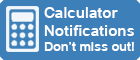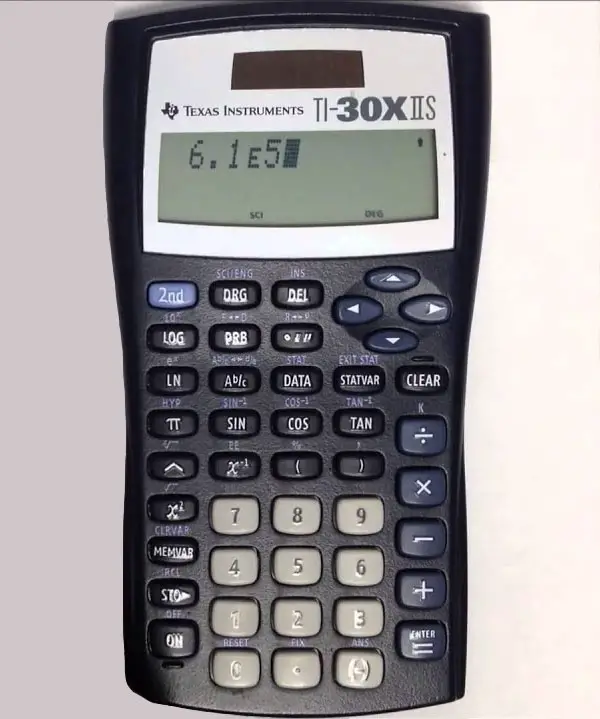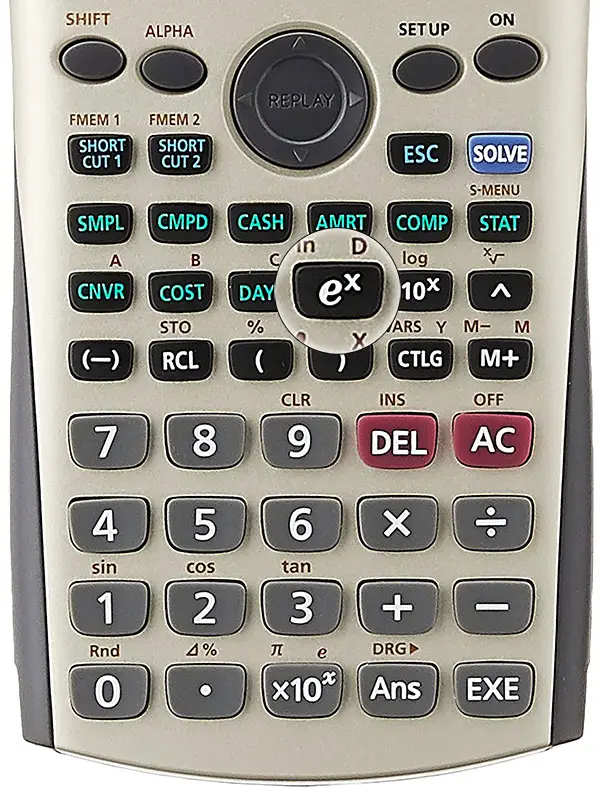Calculator Library
Free Online CalculatorsHome » 87 Calculators » Blog » What Does E Mean On A Calculator?

## WHAT DOES E MEAN ON A CALCULATOR?

The letter "E" is used on many calculators in both uppercase and lowercase forms. It can appear on the calculator screen or keypad and, depending on the context, means either scientific notation or Euler's Number.

## Scientific NotationA capital "E" always represents scientific notation and usually appears on a calculator screen. It represents an exponent of 10 and is always followed by a number representing the value of the exponent. Calculators use scientific notation to display numbers that are very large or very small to make them easier to read and display on a screen.

### How Does Scientific Notation Display Large Numbers?

As an example, if you want to multiply one billion times one billion, you would enter 1,000,000,000 x 1,000,000,000, and the answer would be 1,000,000,000,000,000,000. This number is unwieldy, difficult to read and certainly too big to fit on a calculator screen. To solve this problem using scientific notation, calculators express the 0s as a power of ten.

1,000,000,000,000,000,000 expressed in scientific notation is 1 x 1018, with the calculator version displaying 1E+18. This is sometimes expressed with decimals, such as 1.000000E+18 or simply 1E18. You can read these results as 1 with 18 zeros. The same concept applies to tiny numbers with 0.000000000000000001 expressed in scientific notation as 1 x 10-18 and the calculator version displaying 1E-18.

It's essential to remember that calculators sometimes use a lowercase "e" on the screen to represent exponents, which confuses with Euler's number, which is an unrelated concept.

## Euler's NumberA lowercase "e" always represents Euler's Number when it appears on a calculator keypad. However, it can also represent exponents when it appears on the screen.

Here's a quick rule of thumb to tell the correct meaning of a lowercase "e" on a calculator screen. If it appears before an equals sign, it refers to Euler's number. On the other hand, if it appears after an equals sign followed by digits, it refers to an exponent of 10, as explained previously.

Some calculators will have an e key, however the most common use of a lowercase "e" is on the ex key found on most scientific and financial calculators. This is because Euler's number is a fundamental constant when performing these calculations. If you don't see these keys on your calculator, check the secondary functions that can be accessed by pressing a 2nd or fn key first.

### What Is Euler's Number?

Euler's number, also known as "e", is a mathematical constant with the value 2.7182818284 (to 10 decimal places) and is an essential concept in calculus and other branches of mathematics. It was discovered in 1683 by the Swiss mathematician Jacob Bernoulli and named for Leonhard Euler. Like Pi (𝜋), it's an irrational number, meaning it can't be written as a fraction and the decimal runs forever without repeating.

"e" is the base of the natural logarithm and is used for calculations in many diverse fields, particularly those involving exponential growth and decay. This makes it especially useful when, for example, calculating compound interest in the finance industry or radioactive decay in science.

Euler's number has several interesting properties that make it useful in many mathematical contexts. For example, it's the only number such that the value of its own derivative is equal to itself. This means that the slope of the tangent line to the graph of the function y = ex at any point is always equal to the value of the function at that point.

Euler's number is also closely related to the concept of natural logarithms. The natural logarithm of any number x is the power to which the number e must be raised to obtain the value x. In other words, if y = ln(x), then x = ey. This relationship is known as the natural logarithm property of Euler's number.

In addition to its use in calculus and the study of exponential growth and decay, Euler's number is also essential in other branches of mathematics, such as complex analysis, probability theory and geometry. It's used in various applications, including engineering, physics and computer science.

In summary, Euler's number is an essential concept in many branches of mathematics and has several interesting properties that make it useful in many applications.

 Previous Post« What Does AC Mean On A Calculator? Next PostWhat Does RPN Mean On A Calculator? »